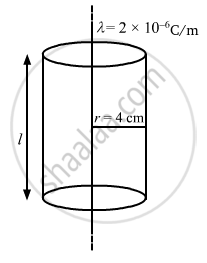Department of Pre-University Education, Karnataka course PUC Karnataka Science Class 12
Share

# Find the Magnitude of the Electric Field at a Point 4 Cm Away from a Line Charge of Density 2 × 10 − 6 C M − 1 . - Physics

ConceptElectric Field Introduction of Electric Field

#### Question

Find the magnitude of the electric field at a point 4 cm away from a line charge of density 2 × 10-6 Cm-1.

#### Solution

Given:
Charge density of the line containing charge, λ = 2 × 10-6 C/m-1

We need to find the electric field at a distance of 4 cm away from the line charge.
We take a Gaussian surface around the line charge of cylindrical shape of radius r = 4 ×10-2 m and height l.The charge enclosed by the Gaussian surface, qen = λl
Let the magnitude of the electric field at a distance of 4 cm away from the line charge be E.

Thus, net flux through the Gaussian surface, phi = oint  vec"E" . vec"d.s"
There will be no flux through the circular bases of the cylinder; there will be flux only from the curved surface.
The electric field lines are directed radially outward, from the line charge to the cylinder.
Therefore, the lines will be perpendicular to the curved surface.
Thus,

phi = oint  vec"E" . vec "d.s" = "E" oint   "ds"

=> phi = "E" xx 2 pi "r""l"

Applying Gauss's theorem,

phi = (q_"en")/∈_0

"E" xx 2 pi "r""l" =( λ"l")/∈_0

"E" = λ/(2 pi "r" ∈_0)

"E" = (2xx10^-6)/ (2 xx 3.14 xx8.85 xx 10 ^-12 xx 4 xx 10^-2)

E = 8.99 ×  105 N/C

Is there an error in this question or solution?

#### APPEARS IN

Solution Find the Magnitude of the Electric Field at a Point 4 Cm Away from a Line Charge of Density 2 × 10 − 6 C M − 1 . Concept: Electric Field - Introduction of Electric Field.
S